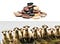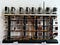# I bought a 500GB HDD. What does that mean exactly?

By Marcos ChiquettoBy Marcos Chiquetto

Let’s go back in time thousands of years, to when humans began to count. Let’s imagine a primitive man wanting to count how many sheep there were in a herd, so he could check if any went missing the next day. To do this, he could maybe use little stones to represent the sheep. For each sheep, he would set a stone apart from the rest. On the next day, all he had to do would be to compare the number of stones with the number of sheep.Counting sheep by means of stones. One stone represents one sheep.

However, instead of stones, our shepherd had other objects that were more readily available: the fingers on his hands. For each sheep, he could stretch one finger.

But what if he ran out of fingers after using his two hands? He could start counting again and again with his fingers, making sure to remember how many times he had restarted, again associating each restart with one finger. This way, the count progresses in rounds of 10, which are also grouped in sets of 10, and so on.

Well, after counting the sheep, our ancient counter would not want to keep his fingers in the same positions until the next morning to check the quantity of sheep. Instead, he would draw a figure representing the fingers. Such figures evolved to become the Arabic numerals we came to use afterwards.

In this article, I’ll skip the long and fascinating history of the zero, one of the most important abstractions conceived by human beings. Instead, I’ll make a huge jump from the ancient shepherd to the numerical system we use today, called “the decimal system”, which uses ten symbols, including zero, to represent ten different basic quantities: 0,1,2,3,4,5,6,7,8,9.

Using numerals instead of the fingers of a hand, it’s easy to register the number of times we restart the count of 10. We just add one additional numeral in front of the first one, representing accumulated groups of ten:

1 group of 10 already counted: 10, 11, 12, 13, 14…

2 groups of 10 already counted: 20, 21, 22, 23, 24…

Someone in a far-off time associated the elements of this counting to the word “digitus” (finger, in Latin). And so, today we call decimal numerals digits.

Much more recently, around 1940, driven by the World War II effort, scientists from Germany, The United States and England focused on projects for powerful electronic machines capable of performing calculations (which the North Americans called “electronic computers”, see our article on the first computers).

Initially, they tried building machines that operated using the decimal system, but they realized this would be too complicated, since electronic circuits don’t have anything similar to hands with 10 fingers. To represent decimal numerals in an electronic machine we would have to use, for instance, 10 different levels of voltages in the circuit. Operating with this kind of data would be an engineering nightmare.

Within the scope of electronic resources, the most natural way of counting things would be something like a lamp: to register a sheep, turn on a lamp. The second level of counting would be a second lamp, and so on. Of course, one would need many lamps, but this wouldn’t make the system complex. Even with many lamps, the system remains simple. Much simpler than the decimal system.

The next figure shows how we can count sheep using a row of lamps. You may assume we have as many lamps as needed.

I will not try to explain the logic of this counting here, since the explanation would be more complicated than the logic itself. I suggest you spend some time observing the sheep and the lamps. It is likely that you will see the rule.

And so, a new system was developed for counting, in which the counting device has only two states (lamp), instead of ten (our hands). As opposed to the decimal system, this system was called “the binary system”. The corresponding set of numerals only needs two symbols. Borrowing from the decimal numerals, the symbols for the binary system are “0” and “1”.

Looking at the above figure, we can create a table relating the first decimal and binary numbers:

Although this numeric system might seem complicated to us because we are used to decimal numbers, it is actually much simpler. This invention paved the way for the development of computers.

Even though it does not derive from the use of fingers, the binary system kept the word “digit” to refer to its symbols, which are called “binary digits”. Moreover, any equipment using this system is referred to as “digital”. Thus, digital equipment is any device that represents quantities using binary digits.

Each binary digit is called a “bit” (abbreviation of binary digit).

But what physical devices are used to identify these two states in a computer? Of course, a computer would not use lamps, as the machine does not need visual information to operate. The very first computers used vacuum tubes, which are electric valves that can open or close the flow of electric current, working much like water faucets. For example, a closed valve means “0” and an open valve means “1”.A logic unit of the IBM series 700 computer, which was launched in 1952. This unit contained 8 vacuum tubes, each one corresponding to one bit. The full machine contained many thousands of vacuum tubes.

Today, bits are stored in microscopic devices, built into silicon wafers through chemical reactions, which form chips. One chip of a few square millimeters can store billions of binary digits.

However, besides numbers, computers also operate with letters and graphic symbols. How can we represent so many elements with just two symbols?

The solution was to gather symbols in groups.

In the early stages of computer development, scientists concluded that groups of 8 binary digits generated a number of combinations large enough to represent the numerals and all letters and graphic symbols. The group of 8 digits was thus adopted as the basic element of information in computers.

A group of 8 bits was called a “byte”. Here are a few examples of bytes:

Comparing this table with the previous one, you will see that the numerals are the binary numbers with a series of digits “0” added to complete groups of 8 digits. The other combinations are used for letters, graphic symbols etc.

Besides letters and numbers, screen pixels and instructions for programming in machine languages are also represented by bytes. So, letters, numbers, graphic content and programming instructions are stored in a computer in bytes.

The previous picture showing vacuum tubes depicts a logic unit which stores one byte (8 bits).

Well, returning to the initial question of this post, what does a 500GB HDD mean?

The international system of units adopts the following multiples:

k (kilo) = 1000

M (mega) = 1,000,000 (million)

G (giga) = 1,000,000,000 (billion)

T (tera) = 1,000,000,000,000 (trillion)

These multiples are powers of 10, appropriate for the decimal system of numerals. In the binary system, powers of 10 don’t have any special meaning. What’s relevant are powers of 2. However, since we are used to thinking in powers of 10, engineers adopted some powers of 2 that give very similar results to these multiples, so that we can evaluate quantities the same way we are used to.

For example:

This is a power of 2 which is very close to 1000. This quantity of bytes is called 1 kilobyte. For practical purposes, we can think of it as being one thousand bytes without much error. The same way, there are other powers of 2 which are very near to one million, one billion, etc, which are named as the other decimal multiples.

So, this is the rule:

1 KB (kilobyte) is approximately one thousand bytes

1 MB (megabyte) is approximately one million bytes

1 GB (gigabyte) is approximately one billion bytes

1 TB (terabyte) is approximately one trillion bytes

So, when you buy a 500 GB HDD, it means you can store approximately 500 billion bytes in it.

Just for comparison, the circumference of the planet Earth measures 49 thousand kilometers. Measured in millimeters, that would be 49 billion millimeters. In other words, a 500 GB HDD stores bytes in a quantity 10 times greater than the circumference of the Earth stated in millimeters.

Marcos Chiquetto is an engineer, physics teacher, translator, and writer. He is the director of LatinLanguages, a Brazilian translation agency specialized in providing multilingual companies with translation into Portuguese and Spanish.